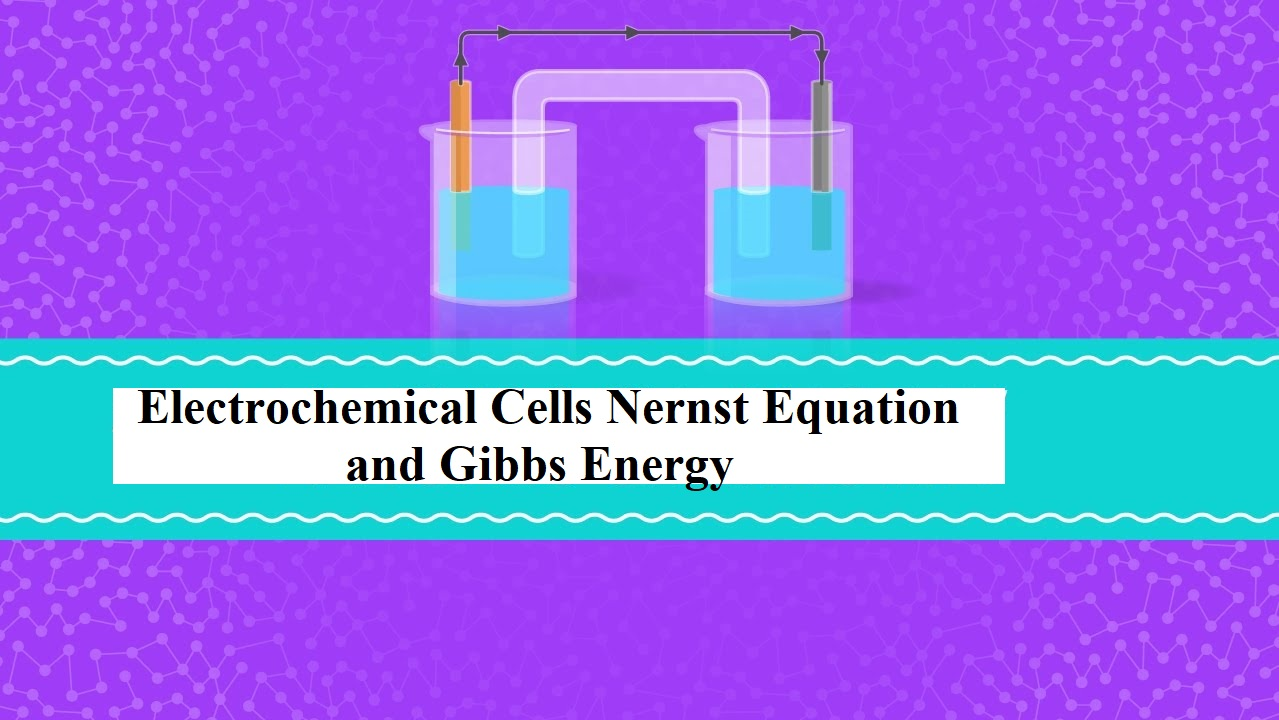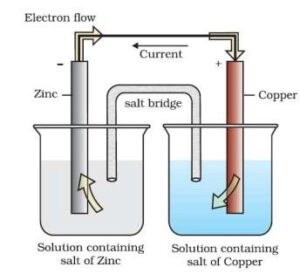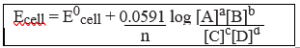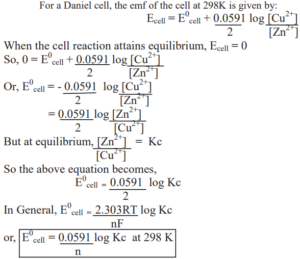# Electrochemical Cells Nernst Equation and Gibbs EnergyElectrochemical Cells Nernst Equation and Gibbs Energy

Syllabus:-

• Electrolytic cells & Galvanic cells
• The function of Salt Bridge
• Redox reaction, EMF of the cell, standard electrode potential
• Standard hydrogen electrode (SHE)
• Nernst equation and its application to Chemical cell
• Equilibrium Constant from Nernst Equation
• Electrochemical Cell and Gibbs Energy

Electrochemistry: It is a branch of chemistry that deals with the relationship between chemical energy and electrical energy and their interconversions.

Redox Reactions: Oxidation is the process that involves the loss of electrons & reduction is a process in which it involves the gain of electrons. The reactions which involve both that reaction simultaneously are called a redox reaction.

Electrochemical Cells: These are devices that convert the chemical energy of some redox reactions to electrical energy. They are also called Galvanic cells or Voltaic cells. An example for a Galvanic cell is Daniel cell.

It is constructed by dipping a Zn rod in ZnSO4 solution and a Cu rod in CuSO4 solution. The two solutions are connected externally by a metallic wire through a voltmeter and a switch and internally by a salt bridge.

A salt bridge is a U-tube containing an inert electrolyte like NaNO3 or KNO3 in a gelly like substance. The functions of a salt bridge are:

1. To complete the electrical circuit
2. To maintain the electrical neutrality in the two half cells.
• Cu2+ + 2 e → Cu(s) (reduction half reaction)
• Zn(s) → Zn2+ + 2 e (oxidation half reaction)These reactions occur in two different portions of the Daniel cell. The reduction half-reaction occurs on the copper electrode while the oxidation half-reaction occurs on the zinc electrode. These two portions of the cell are also called half-cells or redox couples. The copper electrode may be called the reduction half-cell and the zinc electrode, the oxidation half-cell.

Electrochemical Cells Nernst Equation and Gibbs Energy

Electrode Potential: This tendency of a metal to lose or gain an electron when it is in contact with its own solution is called electrode potential.

Standard electrode potential (E0): The electrode potential measured at standard conditions.ie at 298K,1 atm pressure, and at 1 molar concentration.

Standard hydrogen electrode (SHE): The reference electrode used to measure single electrode potential. Its potential is assumed to be zero. It consists of a platinum wire dipped in HCl of 1 molar concentration. Hydrogen gas at 1 atm. is passed through the solution. The electrode can be represented as Pt, H2 /H+(1M).

Nernst Equation For A Cell Reaction:-
Let us consider a general equation
aA   +   bB →  cC   +   dD
E = E0cell  –   2.303 RT × log [C]c [D]d
nF                 [A]a [B]b

Where E0 is the standard electrode potential,
R is the gas constant (8.314 JK–1 mol–1)
F is Faraday constant (96500 C mol–1)
T is the temperature in Kelvin.
Nernst equation can be written as:
Putting the above values Nernst equations can be written asEquilibrium Constant from Nernst Equation:Electrochemical Cells Nernst Equation and Gibbs Energy

Electrochemical Cell and Gibbs Energy of the Reaction:
Electrical work done in one second is equal to electrical potential multiplied by total charge passed. The reversible work done by a galvanic cell is equal to a decrease in its Gibbs energy and therefore if the emf of the cell is E and nF is the amount of charge passed and ∆G is the Gibbs energy of the reaction, then
∆G = –  nFEcell
If the concentration of all the reacting species is unity, then Ecell = E0cell and we have
∆G0 = – nFE0 cell
Thus, from the measurement of E0cell, we can calculate the standard Gibbs energy of the reaction.